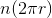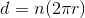# AP Physics B : Understanding Linear-Rotational Equivalents

## Example Questions

### Example Question #24 : Newtonian Mechanics

A wheel of radiusrolls along a flat floor and makesrotations over a period of time. What distancehas the wheel traveled?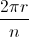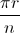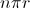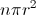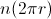Explanation:

In order to find the distance the wheel travels, we need a way to convert angular displacement to linear displacement. We know that the circumference of a circle (or a wheel, in this case) is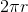. This means that in one rotation, the wheel would travel a distance equal to its circumference.

Distance of one rotation equals:.

Since the wheel travelsrotations, the total distance that the wheel travels will be equal to the distance traveled by one rotation multiplied by the number of rotations.

Distance ofrotations equals: